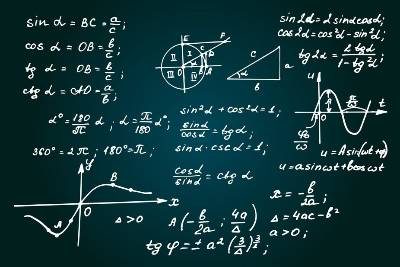# In-Person and Online

GLAs are self-paced computer tutorials that are a great way to reinforce learning and make sure that you are retaining the material you are studying. Tutors are available during the GLA to help you with any questions or challenges you have during the process, and they'll review your work when you are done.

All TLC services require your COC ID Number.• Absolute Values

Compound Inequalities

Decimals: Multiplication and Division

Decimals/Fractions Conversions

Exponent Properties

Exponential and Logarithmic Equations

Exponential Functions

Fractions and Mixed Numbers

Fractions: Multiplication and Division

Graphs/Functions: Analyze

Graphs/Functions: Transformations

Inverse Functions

Linear Equations: Addition and Multiplication Properties of Equality

Linear Functions - Lines

Linear Functions - Slopes

Linear Functions: Forms

Linear Functions: Graphing

Linear Systems – Substitution

Linear Systems: 3 Equations × 3 Variables

Linear Systems: Graphing

Logarithm Basics

Logarithmic Functions

Math Anxiety

Mixed Numbers: Multiplication and Division

Monomials: Multiplying and Simplifying

Operations with Integers

Order of Operations

Polynomials: (+)(−)(×)

Polynomial Division

Proportions

Rational Equations

Rational Exponents

Rational Expressions: Multiplication and Division

Root Functions (graphing)

Scientific Notation

Unit Conversions

Word Problems: Mixture

Word Problems: Percent and Interest

Word Problems: Algebraic

Word Problems: Motion• Analyzing Literary Quotes

Annotation

APA 1

APA 2

Avoiding Plagiarism

Comma Use

Fragments

MLA 1

MLA 2

Prewriting

Run-Ons

Thesis Development

Test Taking Strategies